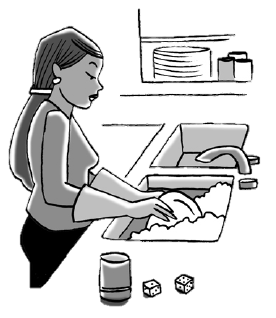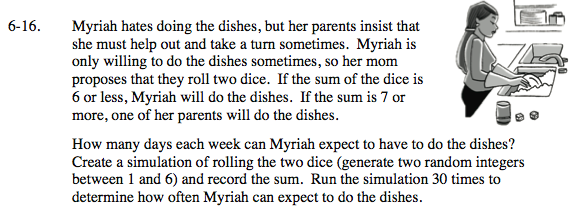### Home > INT3 > Chapter Ch6 > Lesson 6.1.2 > Problem6-16

6-16.
1.Myriah hates doing the dishes, but her parents insist that she must help out and take a turn sometimes. Myriah is only willing to do the dishes sometimes, so her mom proposes that they roll two dice. If the sum of the dice is 6 or less, Myriah will do the dishes. If the sum is 7 or more, one of her parents will do the dishes.

2. How many days each week can Myriah expect to have to do the dishes? Create a simulation of rolling the two dice (generate two random integers between 1 and 6) and record the sum. Run the simulation 30 times to determine how often Myriah can expect to do the dishes. Homework Help ✎Use a random number generator to generate 60 random integers from 1 to 6.

Record the sum of each pair of numbers (60 numbers is 30 pairs).

How many pairs of numbers have a sum of 7 or more?